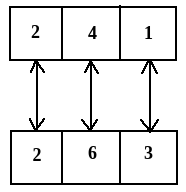# What will be the number at the bottom, if 5 is at the top; the two positions of the dice being as given below:Option 1)   1   Option 2)   2   Option 3)   3   Option 4)   5   Option 5)   6

In the above three diagrams,

To find out the opposite faces we will wright all the elements in the clockwise direction starting with the common element in any two figure.

So taking figure (i) & figure (ii) for the above experiment,Here in this diagram we know that 4 is opposite to 6 & 1 is opposite to 3.

Then numbers left = 2 & 5

So we can say that number opposite to 5 = 2

Exams
Articles
Questions